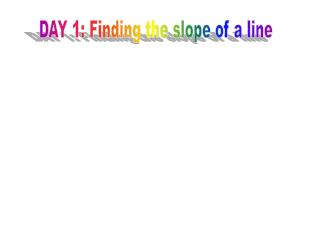DownloadDownload PresentationDAY 1: Finding the slope of a line

# DAY 1: Finding the slope of a line

Télécharger la présentation## DAY 1: Finding the slope of a line

- - - - - - - - - - - - - - - - - - - - - - - - - - - E N D - - - - - - - - - - - - - - - - - - - - - - - - - - -
##### Presentation Transcript

1. DAY 1: Finding the slope of a line

2. Plot points A and B. • A(-2, 3) B(6, 8) • Connect the points. • Find the SLOPE • of the line. Slope “m”

3. Graphing Linear Equations A(-2, 3) B(6, 8) A(x1, y1) B(x2,y2)

4. Graphing Linear Equations • 2. Plot points A and B. • A(5, -3) B(-1, 7) • Connect the points. • Find the SLOPE • of the line.

5. Graphing Linear Equations A(5, -3) B(-1, 7) A(x1, y1) B(x2,y2)

6. 3. Plot points A and B. • A(9, -6) B(9, 5) • Connect the points. • Find the SLOPE • of the line.

7. A(9, -6) B(9, 5) A(x1, y1) B(x2,y2)

8. 4. Plot points A and B. • A(-7, 3) B(5, 3) • Connect the points. • Find the SLOPE • of the line.

9. A(-7, 3) B(5, 3) A(x1, y1) B(x2,y2)

10. What is the slope of the line that passes through (-2, 5) and (6, -10)? • 15/8 • 8/15 • -15/8 • -8/15 [Default] [MC Any] [MC All]

11. What is the slope of the line that passes through and ? • -335/1484 • 335/1484 • 1484/335 • -1484/335 [Default] [MC Any] [MC All]

12. Properties of Slope

13. What is the sign of the slope of the graph below?(positive or negative) • positive

14. Practice 5) Find the slope of each line segment.

15. “What Do You Call a Duck That Steals? Page 153

16. DAY 2: GRAPHING LINEAR EQUAITONS IN SLOPE-INTERCEPT FORM y = mx + b

17. What do you think is the sign of the slope? +m , -m, 0m or

18. What is the slope of the given graph? • undefined

19. What kind of SLOPE will you have?

20. What is the direction of the line with a slope of -2/5? (left or right) • left

21. Slope is the steepness of the line y-intercept = 1

22. Slope is the steepness of the line y-intercept = -8

23. Slope is the steepness of the line y-intercept = origin

24. Slope is the steepness of the line y-intercept = -3

25. Graphing Lines Kutasoftware worksheet

26. Whom Should You See At the Bank If You Need To Borrow Money? Page 155

27. DAY 3: Writing Linear Equations in Slope-intercept Form Express the following linear equations in y-intercept form. y = mx + b

28. Standard Form:

29. Standard Form:

30. Standard Form:

31. Standard Form:

32. 6. 2x + 5y = 30 • m=-2/5 y-intercept=30 • m=2/5 y-intercept=30 • m=-2/5 y-intercept=6 • m=2/5 y-intercept=-6 [Default] [MC Any] [MC All]

33. According to Some Students, What is the True Purpose of Homework? Page 156

34. Y-intercept Form is y = mx + b 1. What is the equation of the line with a slope of 7 and y-intercept at 2?

35. 2. What is the equation of the line with a slope of 2/3 and y-intercept at 5?

36. Y-intercept Form is y = mx + b 3. What is the equation of the line with a slope of -6 and y-intercept at -3?

37. 4. What is the equation of the line with a slope of -1/2 and y-intercept at 5?

38. 5. What is the equation of the graph?

39. \$5-QUESTION 5. What is the equation of the graph?

40. 6. What is the equation of the graph?

41. 7. What is the equation of the graph?

42. 8. What is the equation of the graph?

43. What Did the Ape Think Of the Grape’s House? Page 154

44. Day 4: Writing Linear Equations in Point-slope Form

45. Equation of the Line Given a slope and a point.

46. What is the slope of the line that passes through (-2, 5) and (6, -10)? • 15/8 • 8/15 • -15/8 • -8/15 [Default] [MC Any] [MC All]

47. What is the slope of the line that passes through and ? • -335/1484 • 335/1484 • 1484/335 • -1484/335 [Default] [MC Any] [MC All]

48. What is the slope of the given graph? • undefined